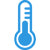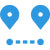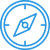### Glossary Of Magnet TerminologyAnisotropic Magnet
A magnet having a preferred direction of magnetic orientation.

Coercive force, Hc
The demagnetising force, measured in Oersteds necessary to reduce the induction, B to zero after a magnet has been previously saturated.Curie temperature, Tc
The transition temperature above which a material loses its magnetic properties.

Flux
The condition existing in a material subjected to magnetising force. The unit of flux is the Maxwell.

Gauss
Lines of magnetic flux per square centimeter. This is a measure of flux density.

Induction, B
The magnetic flux per unit area of a section normal to the direction of flux. This is measured in Gauss.

Intrinsic Coercive Force, Hci
This is a measure of a materials ability to resist demagnetisation. This is measured in Oersteds.

Irreversible Loss
This is defined as the partial demagnetising of a magnet caused by external factors.

Isotropic Magnet
A magnet material whose magnetic properties are the same in any direction.

Magnetic Field Strength, H
A measurement of the magnetic ability to induce a magnetic field at a given point. This is measured in Oersteds.

Magnetic Flux
The total magnetic induction over a certain area.

Magnetizing Force, H
The magnetomotive force per unit length at any point in the magnetic circuit. this is measured in Oersteds.Magnetomotive Force, F
The magnetic potential difference between any two points.

Maxwell
A unit of magnetic flux. One Maxwell is one line of magnetic flux.

Oersted, Oe
A unit of magnetic field strength or magnetizing force.

Orientation Direction
The direction in which an anisotropic magnet should be magnetised in order to optimize the magnetic properties.Saturation
This is a condition where all magnetic moments have become oriented in one direction.

Stabilization
Exposing a magnet to demagnetising influences which are expected in the application in order to prevent irreversible loss during the operation of the magnet.